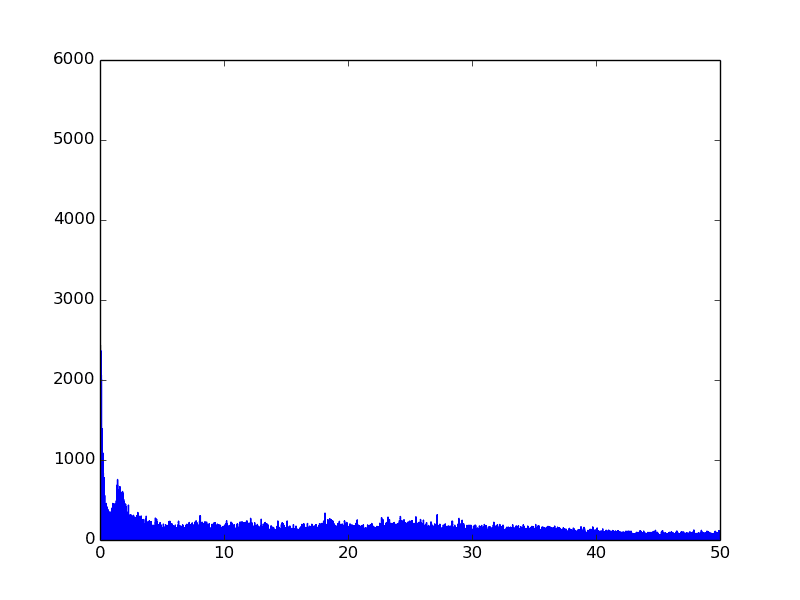# Meaning of having powerful signal near to 0Hz

ramesses
Hello
I computed, with python scipy.rfft, the Fourier transform of signal coming from an accelerometer.
I don't understood what this is the meaning of having a powerful signal near to 0 Hz ?Gold Member
It means that there is a strong DC (or near DC) component to the signal. It may just be a voltage offset, or it may be the acceleration of gravity. It depends on how the signal is coupled and how the circuit is adjusted.

When we analyze many different data sets for the oscillatory components (temperature, tides, sounds) we often take a pre-analysis step to remove the DC or near DC components. Sometimes, it is just subtracting the mean of the whole signal. Other times it may be fitting to a cubic polynomial and then subtracting the cubic polynomial from the original signal and then taking the Fourier transform of the oscillatory components.

In any case, graphs are easier to understand if care is taken to preserve the proper units on both the horizontal and vertical axes (frequency in Hz and acceleration in m/s/s, for example).

•berkeman and donpacino
ramesses
thank you :)
My last question is about band. My sensor frequency is about 100 Hz
Why do I have to use 0~50 Hz in fft ? Why not to use 0~100 Hz ?

Gold Member
thank you :)
My last question is about band. My sensor frequency is about 100 Hz
Why do I have to use 0~50 Hz in fft ? Why not to use 0~100 Hz ?

The highest frequency in an fft is 1/2 the sampling frequency.

If your sensor is sensitive to 100 Hz, you can increase your sampling rate to 200-1000 Hz and see higher frequencies.

ramesses
I have a sampling of 30 minutes with my sensor with 100 Hz. and I want to reduce eliminate signal in [25~100] Hz.
So what I do is :
I apply for each 100 samples a wavelet of second level.
I put 0 in details, and reconstruct the signal.
Now to see the efficiency, what do I need ?
Is the power spectral density useful in my case ?